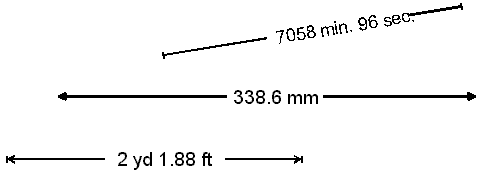# dimensioning

Also found in: Dictionary, Thesaurus, Medical, Wikipedia.

## dimensioning

[də′men·chən·iŋ]
(graphic arts)
Assigning of dimensions to a mechanical drawing.

## Dimensioning

Measurement and placement of dimensional information during the process of drawing the plans, elevations and details.

## dimensioning

In CAD programs, the management and display of the measurements of an object. There are various standards that determine such things as tolerances, sizes of arrowheads and orientation on the paper.

Dimensioning ExamplesReferences in periodicals archive ?
To prove the first part of condition (2), we assume that the dimensioning is proper and we need to show that [Mathematical Expression Omitted]
Since proper dimensioning implies completeness, each bar [H.sub.k] is related by a dimension-set to at least one other parallel bar [H.sub.l], so [Mathematical Expression Omitted] is H, the set of all the horizontal bars.
For the second part of condition (2), we are given that [Mathematical Expression Omitted] and we need to show that the dimensioning is complete.
The normalon proper dimensioning theorem can be rephrased more compactly as the following corollary:
A normalon of rank r is dimensioned properly if and only if each of its horizontal and vertical dimensioning graphs is an r node tree.
The requirement that the graph be a tree takes care of the nonredundancy of the dimensioning, while the requirement that the tree has exactly r nodes guarantees the dimensioning's completeness.
The proper dimensioning theorem provides a sound theoretical basis for a variety of operations in the view-level analysis phase of engineering drawing understanding.
Figure 9 is an example of an improper dimensioning of the normalon of Figure 4, in which only the first condition is met.
Furthermore, if k = 1, then the dimensioning graph consists of two disconnected components, and the search can be guided by the fact that the missing dimension-set should denote the distance between a bar in one component of the graph and a bar in the other component.

Site: Follow: Share:
Open / Close